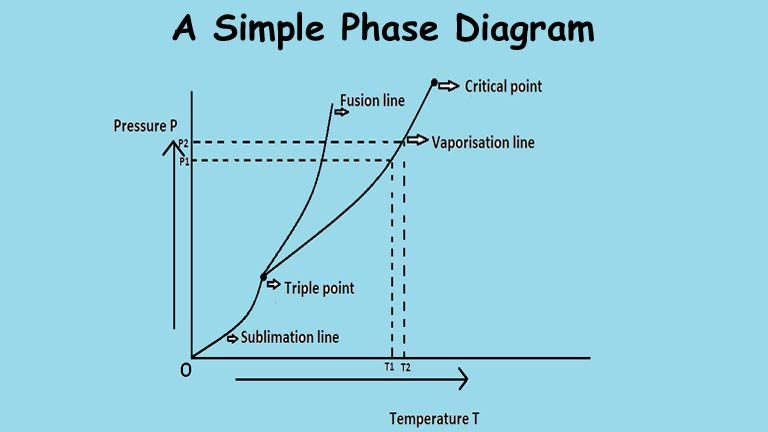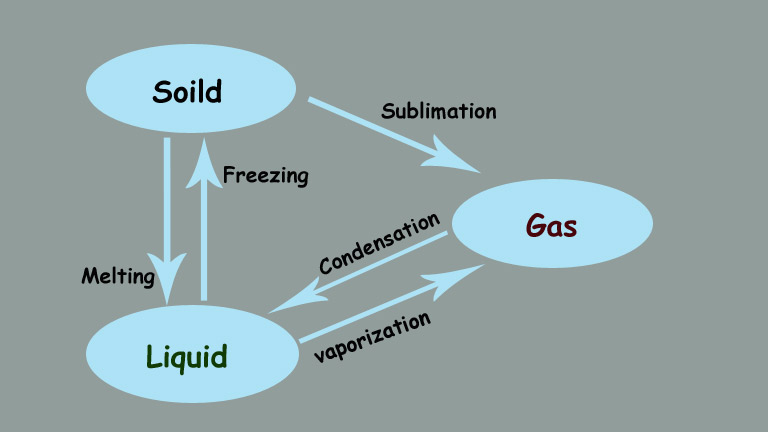# Latent Heat definition, example, unit, latent heat of water, fusion, vaporization

0
20324## Latent Heat definition

The substance changes its phases like Solid, Liquid & Gases. In this process when a substance or body melts or evaporates, some heat is released or absorbed in the form of thermal energy during constant temperature is called Latent Heat.

Order of Phase transitions changes: There are many orders of phase changes.A Phase diagram is a graph between Temperature (T) and pressure (P) in between the equilibrium state of any two phases of a substance.

The First Order of phase change transition: Where the heat is either absorbed or released.

In other words, we can say that where a system either released or absorbed a fixed amount of energy.

Examples: Latent Heat is usually a first-order phase change transition.

The first-order phase transition should have a change in volume and entropy.

For the first-order phase transition, the first-order derivative of the Gibbs function change discontinuously.

As you know first-order phase transition occurs between the triple point and critical point.

### What is a Triple point in thermodynamics?

In thermodynamics, the triple point is a point on a Pressure-temperature (P-T diagram corresponding to temperature and pressure under which a substance can co-exist in equilibrium in all three phases of materials.

Phases of materials are Solid, liquid, gases states. The triple point is always unique.

The triple point of water: 273.16 K at a pressure of 611.2 Pascals or (0.006 atm).

### What is a Critical point in thermodynamics?

In thermodynamics, the critical point is the endpoint of the phase equilibrium curve.

In other words, we can say that it the point or state at which two phases of a substance initially become distinguishable from one another.

The critical point of water: 647 K at a pressure of 22.064 MPa or (218 atm).

Related Topic – Complete Carnot Cycle, efficiency, PV diagram, TS diagram, Theorem, Derivation, heat engine

## Order of phase transitions in latent heat.

The Second-order phase change transition: In this transition, there is no absorption or release of heat energy.

Examples: Bose-Einstein condensation, Liquid helium below a specific temp (around 2.19K) can exist in two different phases, named helium I and helium II, ferromagnetic transition, etc.

### What is Transition temperature?

It is the temperature at which phase change takes place.

Glass transition temperature (Tg): It is the temperature a which an amorphous polymer material generally change their state from glassy to rubbery as the temperature is increased.

Examples are given below,

In latent heat, the kinetic energy of particles in a substance remains the same and the energy which is absorbed or release comes from the potential energy in between bonds of particles.

### What is the heat of vaporization?

When a substance changes from liquid to gas state.

### What is the heat of fusion?

When a substance changes from liquid to solid state (freezing) or melting a solid.

### What is the heat of sublimation?

When a substance changes its state direct from solid to vapors.

### What is the heat of Condensation?

When a substance changes from gases to a liquid state.### What are the Units of Latent Heat?

Latent heat is measured in Cal g-1  in the C.G.S system and in the SI system the units measured in J kg-1 .

## What is the Latent heat formula?

The formula of latent heat is given by,

Q = mL or L=Q/m

Where L = latent heat

Q = amount of heat

m = mass of the substance.

### Simple examples of latent heat

When we boil the water and the temperature remains at 100°C until the last drop evaporates,

due to the latent heat of vaporization, the added heat in the water is absorbed and carried away by releasing vapor molecules.

Related Topic – Liquefaction of gases and its Methods, Applications, Examples, Principal, Linde-Claude, Co2, Hydrogen

### What is the latent heat of fusion of water?

That is 334kJ/kg.

### What is the latent heat of vaporization of water?

That is 2264.705kJ/kg.

Let us calculate latent heat by a simple expression: Determine the latent heat of a 10kg substance if the amount of heat for a phase change is 200k.cal.

By using the formula,

Given that Mass (M) = 10 kg,   Amount of heat (Q) = 200k.cal.

Latent heat = Q/M = 200/10 = 20k.cal. kg-1

Related Topic – 7 Differences between Joule Thomson effect and Adiabatic expansion

## Equations in latent heat

There are two latent heat equations, Equation first, and equation second.

### First latent heat equation

The first latent heat equation which is also, named as Clausius-Clapeyron equation is,

dP/dT = L/T(Δv)       Where Δv = v2-v1

dP/dT = L/T(v2-v1)

This equation deals with the relationship between pressure and temperature for the condition of equilibrium between the two phases.

The two phases could be vapor and solid for sublimation or liquid and solid for melting.

### The second latent heat equation

C2−C1 = dL/dT− L/T

Here the symbols C2 and C1 are used for specific heats, and T and L are respectively for temperature and latent heat.

#### Table for different phase changes quantities:

 Substance Lf (kJ/kg) Tb (°C) Tm (°C) Lv (kJ/kg) Water 334 100 0 2264.705 Ammonia 332.17 -33.34 -77.74 1369 Helium -268.93 21 Hydrogen 58 -253 -259 455 Methane 58.4 -164 -182 112 Propane 80.1 -44.5 -188 Lead 23 1750 327.5 871 Nitrogen 25.7 -196 -210 200 Oxygen 13.9 -183 -219 213